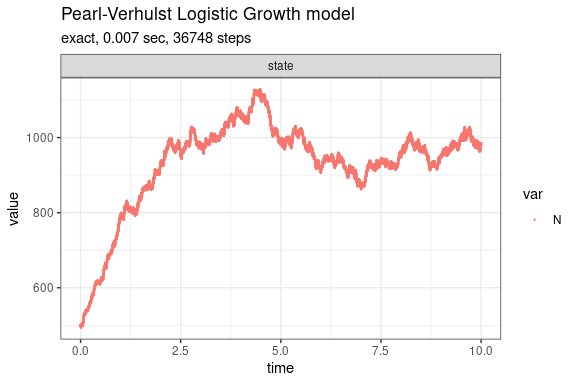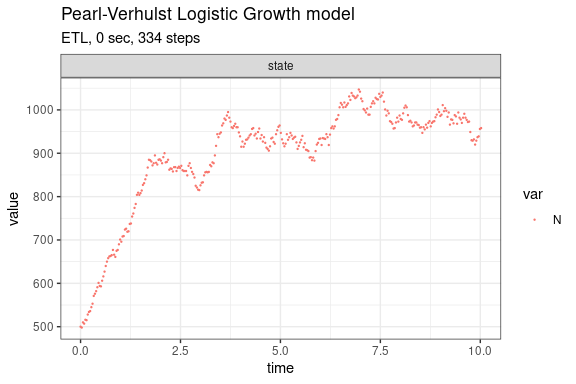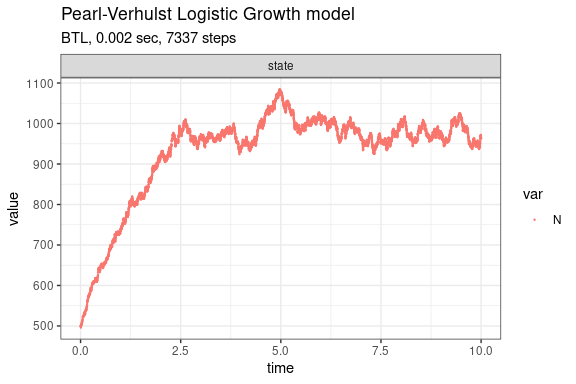# Pearl-Verhulst Logistic Growth model (Kot, 2001)

The logistic growth model is given by dN/dt = rN(1-N/K) where N is the number (density) of indviduals at time t, K is the carrying capacity of the population, r is the intrinsic growth rate of the population. We assume r=b-d where b is the per capita p.c. birth rate and d is the p.c. death rate.

This model consists of two reaction channels,

 N ---b--->  N + N
N ---d'---> 0

where d'=d+(b-d)N/K. The propensity functions are a_1=bN and a_2=d'N.

Define parameters

library(GillespieSSA2)
sim_name <- "Pearl-Verhulst Logistic Growth model"
params <- c(b = 2, d = 1, K = 1000)
final_time <- 10
initial_state <- c(N = 500)

Define reactions

reactions <- list(
reaction("b * N", c(N = +1)),
reaction("(d + (b - d) * N / K) * N", c(N = -1))
)

Run simulations with the Exact method

set.seed(1)
out <- ssa(
initial_state = initial_state,
reactions = reactions,
params = params,
final_time = final_time,
method = ssa_exact(),
sim_name = sim_name
)
autoplot.ssa(out)Run simulations with the Explict tau-leap method

set.seed(1)
out <- ssa(
initial_state = initial_state,
reactions = reactions,
params = params,
final_time = final_time,
method = ssa_etl(tau = .03),
sim_name = sim_name
)
autoplot.ssa(out)Run simulations with the Binomial tau-leap method

set.seed(1)
out <- ssa(
initial_state = initial_state,
reactions = reactions,
params = params,
final_time = final_time,
method = ssa_btl(mean_firings = 5),
sim_name = sim_name
)
autoplot.ssa(out)